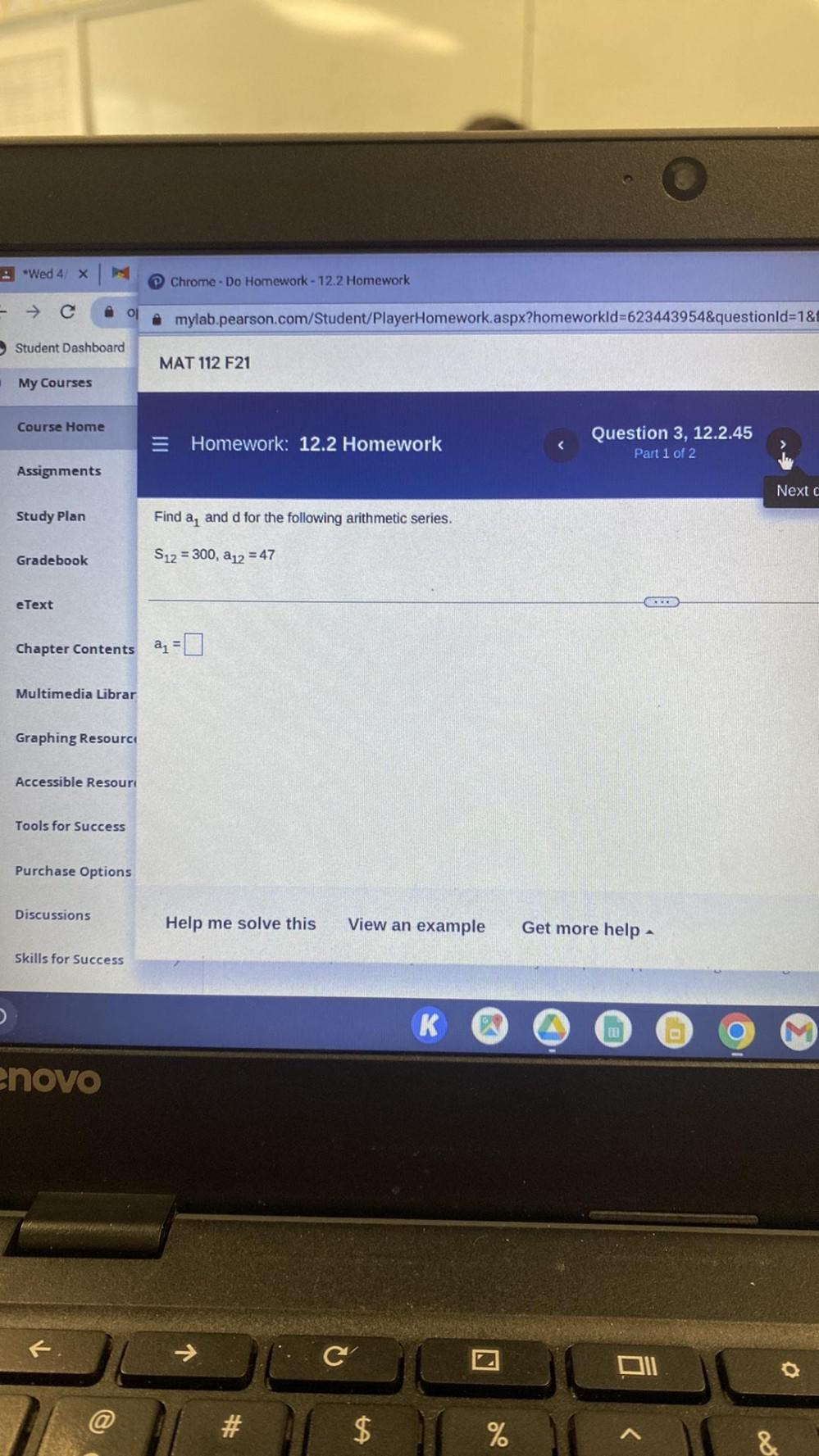Question:

# Find a1 and d for the following arithmetic series. S12 = 300, a12 = 47 a1 = ____.Find a1 and d for the following arithmetic series. S12 = 300, a12 = 47 a1 = ____.